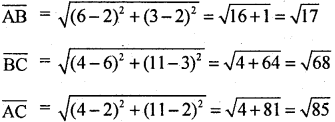# 1st PUC Basic Maths Question Bank Chapter 14 Standard Angles of Allied Angles

Students can Download Basic Maths Chapter 14 Standard Angles of Allied Angles Questions and Answers, Notes Pdf, 1st PUC Basic Maths Question Bank with Answers helps you to revise the complete Karnataka State Board Syllabus and score more marks in your examinations.

## Karnataka 1st PUC Basic Maths Question Bank Chapter 14 Standard Angles of Allied Angles

Question 1.
Find the value of
(i) cosec (-765°)
(ii) sec (-1305°)
(iii) sin 585°
(i) -cosec 765°
= -cosec (360x2 + 45°)
= -cosec 45°
= – √2

(ii) sec (1305°)
= sec (360.3 + 225)
= sec 225°
= sec (180 + 45°)
= -sec 45°
= – √2

(iii) sin (360 + 225°)
= sin 225°
= sin (180 + 45°)
= – sin 45°
= – √2Question 2.
Evaluate: $$\frac { 4 }{ 3 }$$tan2 120° + 3 sin2 300° – 2cosec2240°- $$\frac { 3 }{ 4 }$$cot2 60°
Now tan 120° = tan (180° – 60°)
= -tan 60°
= -√3
sin 300° = sin (360° – 60°)
= – sin 60° = $$\frac{-\sqrt{3}}{2}$$
cosec 240° = cosec (180° + 60°)
= – cosec 60° = $$\frac{-2}{\sqrt{3}}$$Question 3.